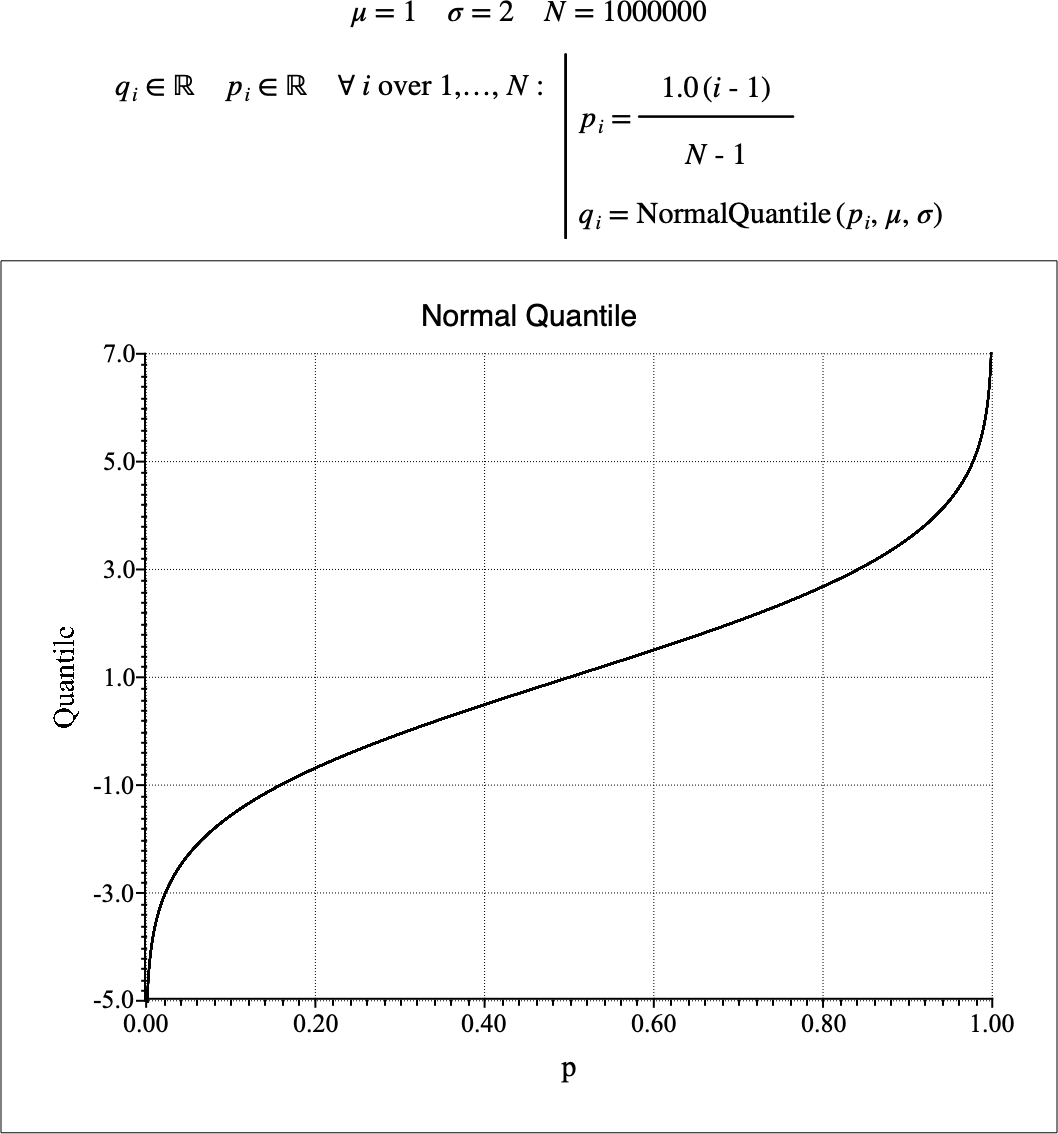# $$\text{NormalQuantile}$$¶

You can use the $$\text{GammaQuantile}$$ function to calculate the quantile function of the normal or Gaussian distribution. The quantile function is the inverse of the cumulative distribution function.

You can use the \normalq backslash command to insert this function.

The following variants of this function are available:

• $$\text{real } \text{NormalQuantile} \left ( \text{<p>} \right )$$

• $$\text{real } \text{NormalQuantile} \left ( \text{<p>}, \text{<}\mu\text{>} \right )$$

• $$\text{real } \text{NormalQuantile} \left ( \text{<p>}, \text{<}\mu\text{>}, \text{<}\sigma\text{>} \right )$$

The $$\text{p}$$, $$\text{<}\mu\text{>}$$, and $$\text{<}\sigma\text{>}$$ are scalar values representing the cumulative probability interest, the mean value of the distribution, and the standard deviation respectively. The $$\text{<}\mu\text{>}$$ and $$\text{<}\sigma\text{>}$$ parameters are optional. If the $$\text{<}\mu\text{>}$$ parameter is not included, a value of 0 is used. If the $$\text{<}\sigma\text{>}$$ parameter is not included, a value of 1 is used. Note that the function is defined over the range $$0 \leq p \leq 1$$ and $$\sigma > 0$$. The $$\text{NormalQuantile}$$ and will generate a run-time error or return NaN for values for which the function is not defined.

The returned value is calculated directly using the relation:

$\text{NormalQuantile} \left ( p, \mu, \sigma \right ) = \mu + \left ( \sigma \sqrt{2} \right ) \text{erf}^{-1} \left ( 2 p - 1 \right )$

Where $$\text{erf}^{-1}$$ represents the inverse error function.

The normal distribution is one of the most widely used distribution as it represents the sum of a large number of random processes with fininte mean and variance. The resulting distribution from the sum will approach the normal distribution as the number of random processes approaches infinity. In practice, only a small number of random processes are needed to obtain a close approximation of the normal distribution.

Figure 179 shows the basic use of the $$\text{NormalQuantile}$$ function.Figure 179 Example Use Of the NormalQuantile Function